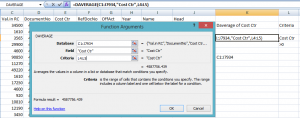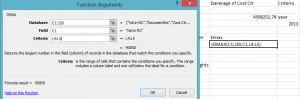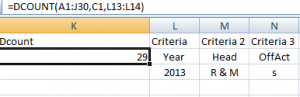# Handy Database Functions in Excel 2013

While working with spreadsheets, we frequently need to retrieve a specific value from large data sets. Sometimes, we need to filter out values on the basis of some criterion. And at other times, we wish to perform a simple arithmetic operation which can be as simple as calculating sum or finding average of a particular column. Today, we will learn the different database functions offered by the excel 2013 to help us to handle bulky spreadsheets effectively.

## 1-DAverage:

As depicted by its name, DAverage computes average of any column in database. It has three arguments: database, field and criteria.

• Database is the entire spread sheet or you may select a part of it.
• Field is the column on which you will apply the average formula. You can refer to a particular column by specifying its number or its name (inside quotation marks).
• Criterion is the basis on which values will be extracted from database and their average will be calculated. It is the range of cells that contains your specified column name and the condition.

In our example, we have calculated the average of ‘Cost Ctr’ column of values in which ‘Cost Ctr’ is greater than zero.  You must be thinking that we can calculate the average by using the simple Average formula, why shall I get into complexity? Let’s consider for a moment, that you need to calculate the average of ‘Cost Ctr’ that was recorded in the Year 2013.  Can you do this with the traditional Average formula?No! And, this is where DAverage function shows its significance. We mention the complete database so that we can calculate the average by filtering the values on the basis of any column included in the database. To achieve the above target, I can write Year as label and 2013 as condition in the criteria cell range.

## 2-DMax and DMin:

DMax returns the largest number from the column in the database, meeting certain conditions. Similarly, DMin returns the smallest number from the column. They have the same arguments like DAverage i.e., database, field and criteria.

In the following example, we have found the maximum value of ‘Val. in RC’ column which corresponded to the ‘Year 2013’. Note that ‘Year’ is another column in the database.## 3-DSum and DProduct:

DSum functions sums up all the values in a particular column of the database which meets a certain criterion. Similarly, DProduct function multiplies them all. The arguments and syntax stays the same as other Database functions.

## 4- DVar and DStDev:

Variance and Standard Deviation are very crucial functions which can not be over looked in any accounting task. DVar and DStDev help you estimate the variance and the standard deviation on a sample from selected database enteries.

## 5- DCount and DCountA:

Sometimes we need to calculate the total number of items present in our inventory. Perceptibly, we can not go through entire database and count the items one by one. So, we make use of the DCount function counts the items in fields or column in the database that match the conditions you specify, using criteria. DCountA performs an opposite task calculating the number of empty cells in the column.

This brings us to the last point yet interesting point of discussion. The strength of these database functions lies on the fact that they can perform filtering of values by checking them against multiple criterions. For doing so, specify the column name and the condition in adjacent columns, so they easily make contiguous cell range. In the following example, we have defined three criterions i.e. how many items in ‘Val. in RC’ were recorded in year 2013, with OffAct ‘S’ and the head were ‘R & M’.We hope that we have added to your knowledge and made the database handling easier for you.